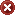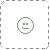String ball object 3D printed by Shapeways using Full Colour Sandstone (it's not actually sandstone; I think it's some kind of nylon derivative). The object was created using Functy and is a couple of parametric curves defined by the following formulae:

(x, y, z) = (40*cos(atan(2*sin(a*10*p)))*cos(2*a*p), 40*cos(atan(2*sin(a*10*p)))*sin(2*a*p), 40*sin(atan(2*sin(a*10*p)))),

(r, g, b) = (1, ((1+sin(4*(p+((1+sin(10*a*p))*8*p))))/2)*0.6, ((1+sin(4*(p+((1+sin(10*a*p))*8*p))))/2)*0.4).

You can also see a 3D render that shows what the object might look like if it were made from glass.Submitted on
August 28, 2012
Image Size
191 KB
Resolution
1620×903
Thumb

Views
243
Favourites
1 (who?)
2
8

### Camera Data

Make
Nokia
Model
N900
Shutter Speed
1/64 second
Aperture
F/2.8
Focal Length
5 mm
ISO Speed
100
Date Taken
Aug 29, 2012, 4:06:03 PM